# The Mother of Artificial Intelligence-Gradient Descent

Gradient Descent has been one of the popular optimization algorithms present in current times. Momentum is its greatest friend. Momentum provided a new perspective to research in optimization. From months, I was trying to understand backpropogation and when I learned, it felt totally overwhelming.

This goes as a tribute to the Mother of Artificial Intelligence which is Gradient Descent.

Here’s a more mathematical explanation of Gradient Descent and its variants → https://colab.research.google.com/drive/1lNhdf4TwPvQrN3CKyGhPmtxC9uOGGKZW#scrollTo=N9jb8SnWyDx1&forceEdit=true&offline=true&sandboxMode=true

### The Birth of the Mother

The first question which comes in most of our minds is that:

Why do we need optimization algorithms? Do they really have uses in economics and mathematics?

Here’s where you will see Mathematical Optimization. Wikipedia says,

In the simplest case, an optimization problem consists of maximizing or minimizing a real function by systematically choosing input values from within an allowed set and computing the value of the function.

In simpler words, a mathematical function is optimized in order to get the minimum or maximum results out of a function. Suppose, if we have a function f( x ) = x²

Now, the minima of this function or a particular point on the line which has the minimum value are (0, 0 ). If x = 0 and then y = 0. You can’t get a value that’s smaller than 0.

Initially, the Random optimization algorithm was used for optimization. It consisted of random picking up a set of values, plugging them in the function, and fetch the results. The smallest result was considered as the minima.

Gradient Descent proposed a newer and efficient way to reach the minima or maxima ( opposite of minima ). It devised the use of gradient or slope of a function which needs to be optimized.

### What is the gradient of a function? ( No calculus guaranteed! )

That’s Calculus

In simpler words, the gradient is the slope of the tangent line to a curve at a specific point. For example, we take our f( x ) = x² function. The gradient could be calculated producing a derivative of the function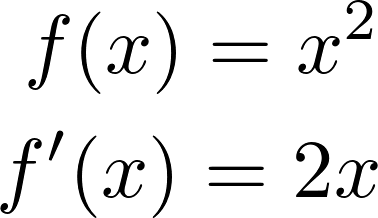Try searching for the power rule if this seems confusing!

For a multivariable function, the gradient is a vector of all the partial derivatives of a function.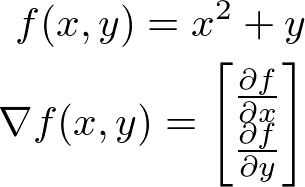∇ symbol stands for the gradient.

### What’s in Artificial Intelligence?

In machine learning or AI, we try to explain every possible relationship in the form of the function which has some parameters and provides some sort of result. For example, we give the AI an image of a cat and train it to label the image as a cat. We can transform this classification task into some function like: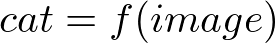Ridiculous function right? That’s ML!

But, how do we find this function? For the function which we used as an example f( x ) = x², we knew that if y in the output and x is the input then the relationship between them is y = x².

How does a computer find such as a relationship with the image and its label “cat”.

#### Universal Function Approximation Theorem

This nice theorem is pretty useful for Artificial Neural Networks. I have written a story on it.

Basically, it states that an Artificial Neural Network with some hidden neurons can approximate any function.

Our Artificial Neural Networks ( ANNs ) can learn to approximate our cat-image function as well. But learning is not enough, learning it thoroughly is needed.

Solve it like a Pro!

Gradient Descent minimizes the objective function. But, what do we need to minimize in ANNs? That’s our loss function: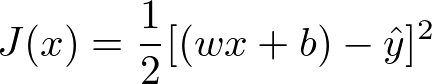A simple loss function for a NN.

We need to minimize the values of w and b so that the value of J decreases and our NN makes nice predictions. Let’s have a look at the Gradient Descent Update Rule: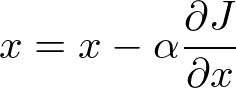Where α is the learning rate or step size. Steps can be called as the strides which our algorithm takes towards the minima. For example, if we need to optimize the w parameter then: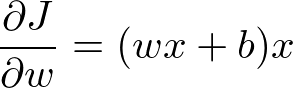See here for steps.

Hence, step by step we are approaching the minima and our NN learns more efficiently.

### That’s all!

Lot’s of Math right? That’s Gradient Descent, the teacher, mother, of intelligence. Thank You and happy AI learning.